Ex 7.3

Chapter 7 Class 8 Comparing Quantities
Serial order wise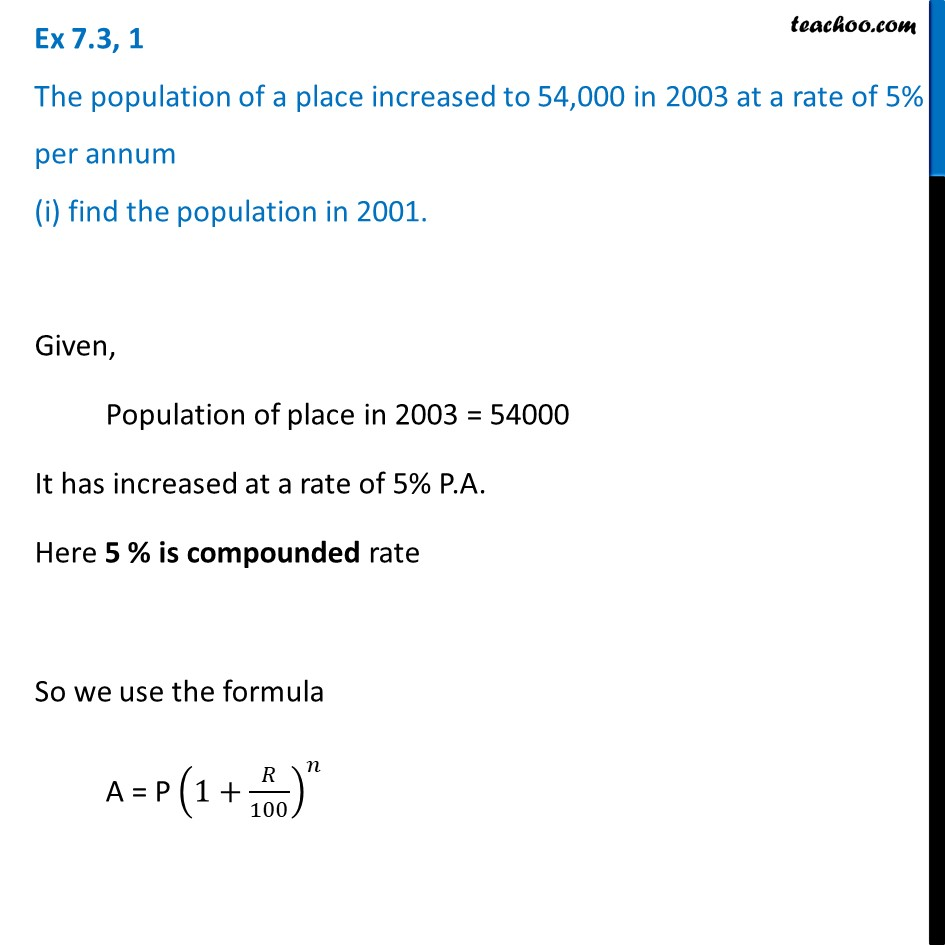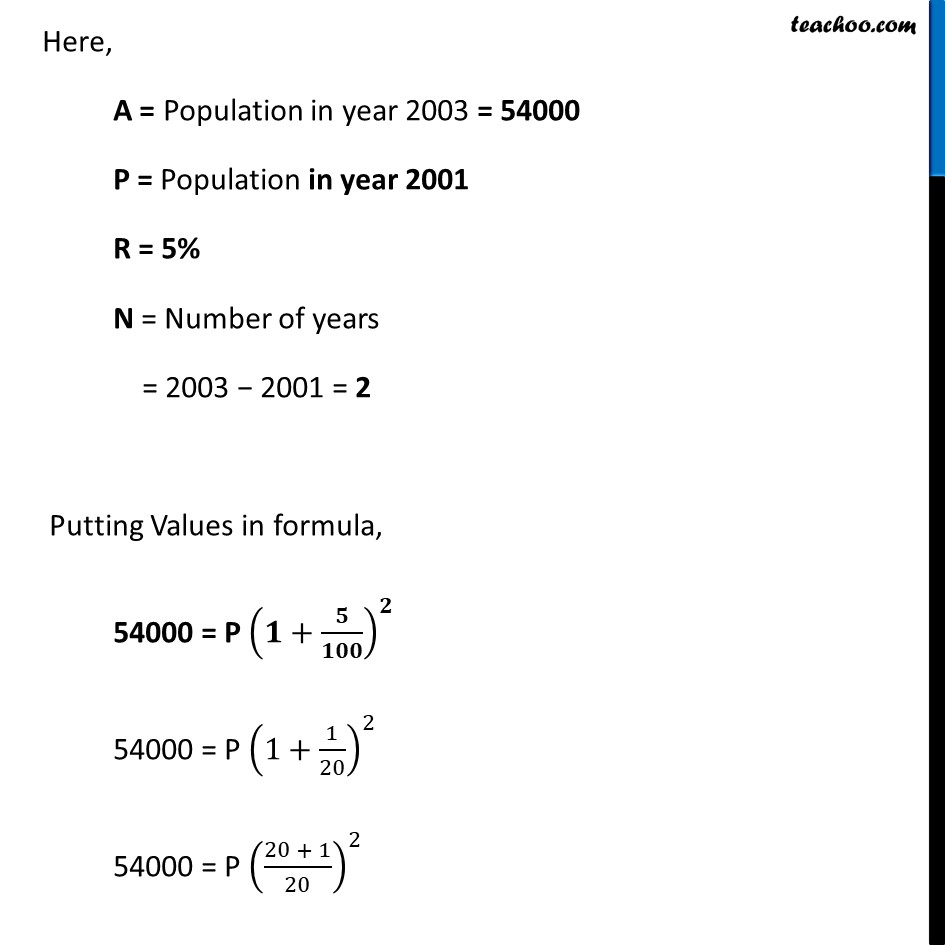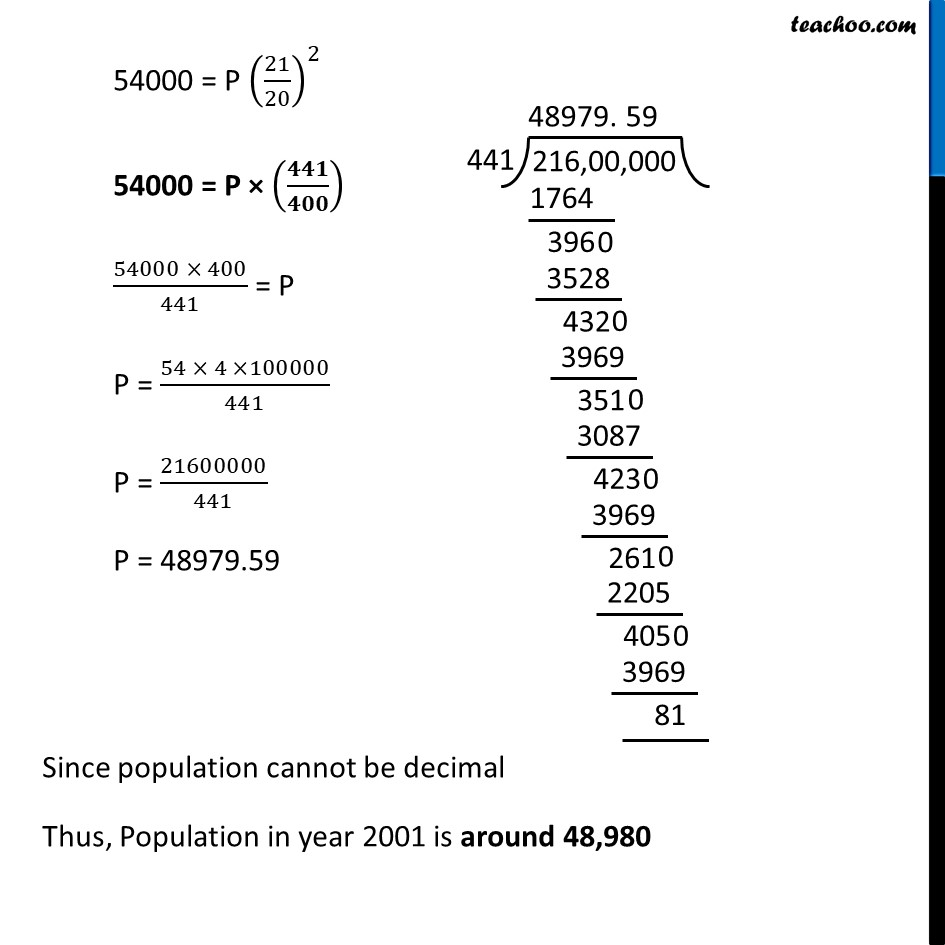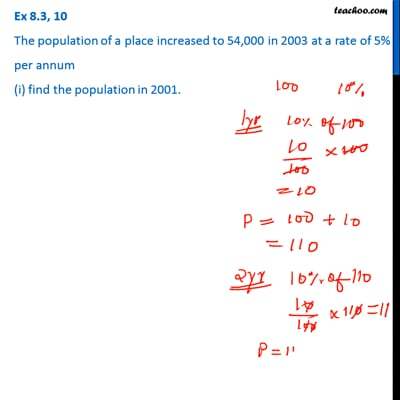This video is only available for Teachoo black users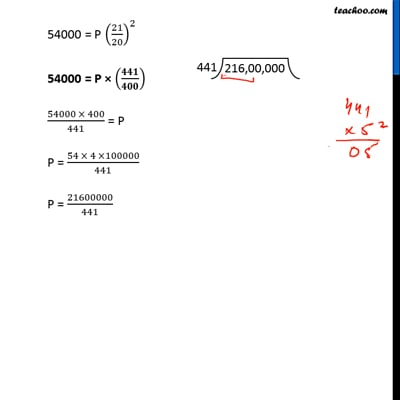This video is only available for Teachoo black users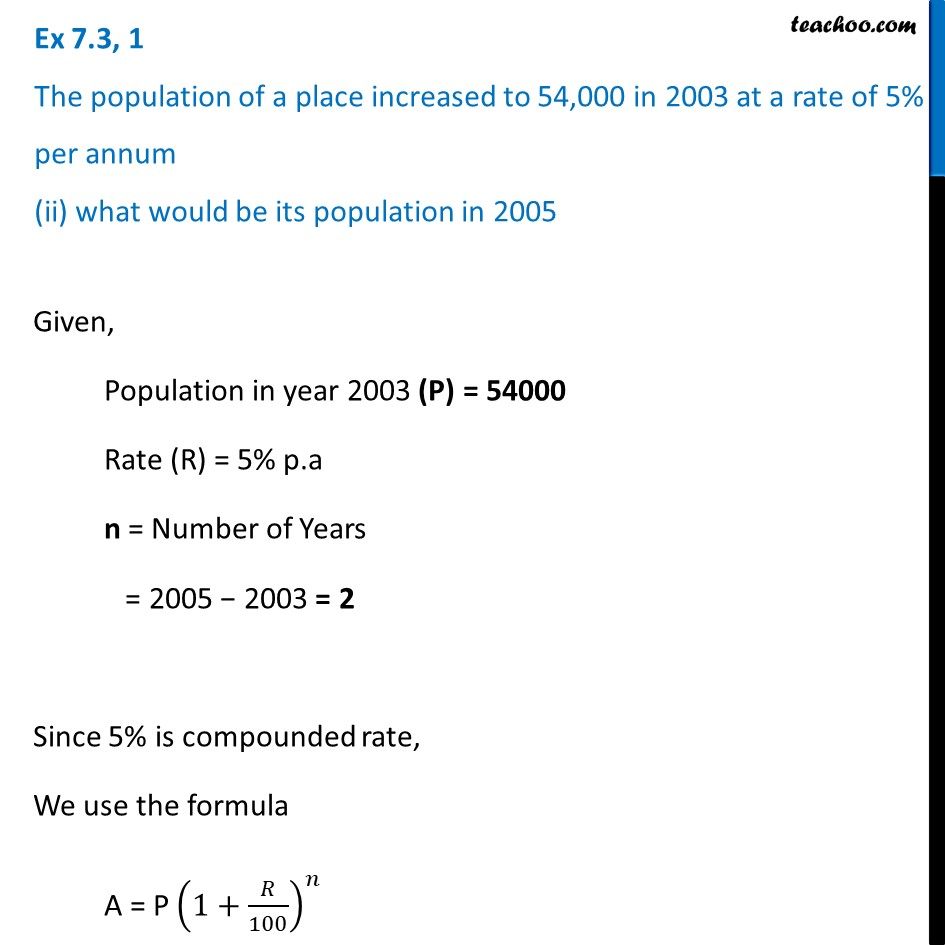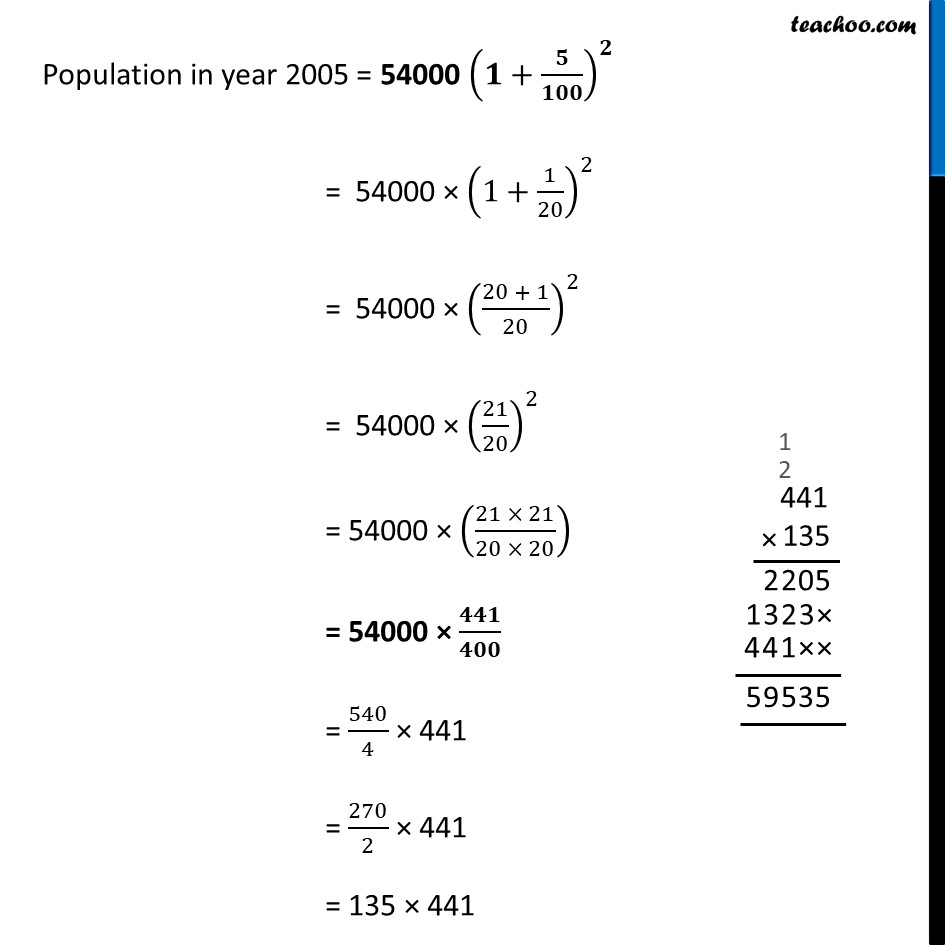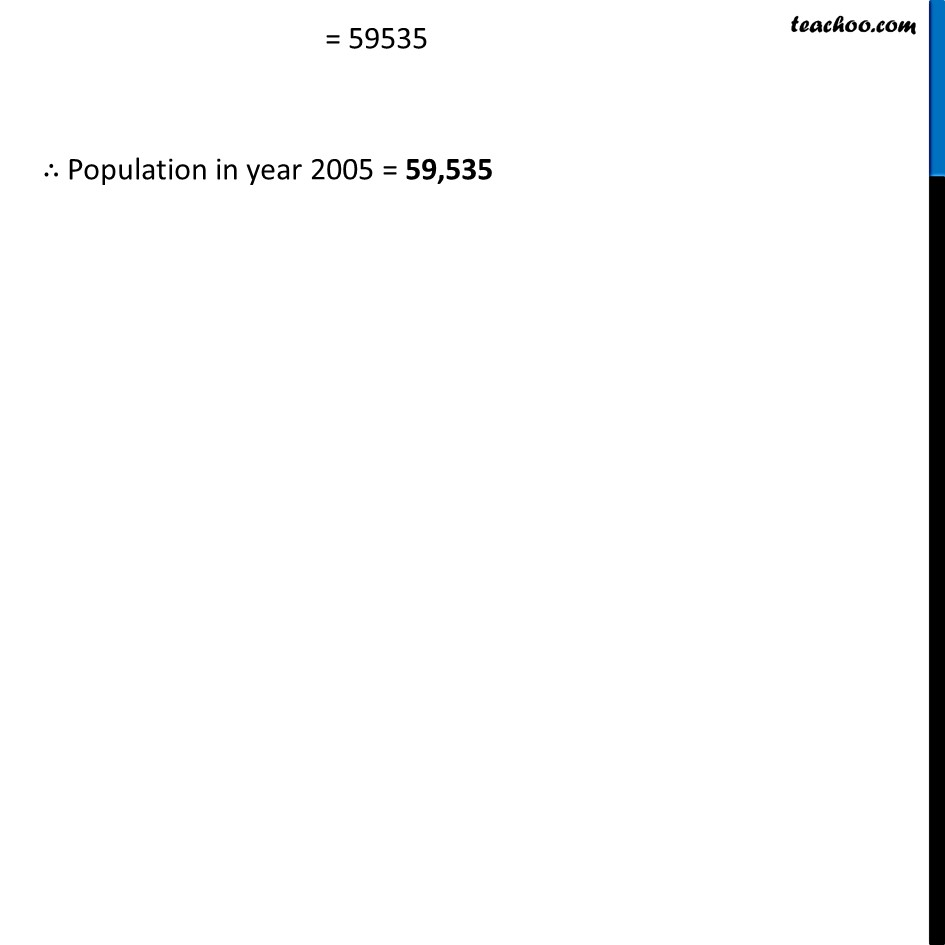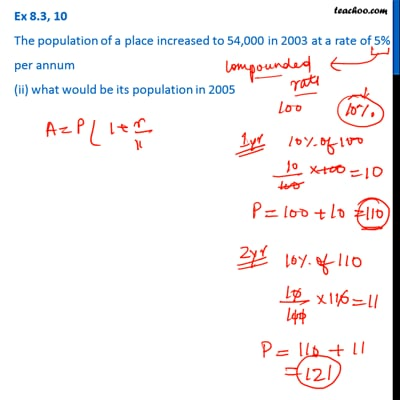This video is only available for Teachoo black users

Learn in your speed, with individual attention - Teachoo Maths 1-on-1 Class

### Transcript

Ex 7.3, 1 The population of a place increased to 54,000 in 2003 at a rate of 5% per annum (i) find the population in 2001.Given, Population of place in 2003 = 54000 It has increased at a rate of 5% P.A. Here 5 % is compounded rate So we use the formula A = P (1+𝑅/100)^𝑛 Here, A = Population in year 2003 = 54000 P = Population in year 2001 R = 5% N = Number of years = 2003 − 2001 = 2 Putting Values in formula, 54000 = P (𝟏+𝟓/𝟏𝟎𝟎)^𝟐 54000 = P (1+1/20)^2 54000 = P ((20 + 1)/20)^2 54000 = P (21/20)^2 54000 = P × (𝟒𝟒𝟏/𝟒𝟎𝟎) (54000 × 400)/441 = P P = (54 × 4 ×100000)/441 P = 21600000/441 P = 48979.59 Since population cannot be decimal Thus, Population in year 2001 is around 48,980 Ex 7.3, 1 The population of a place increased to 54,000 in 2003 at a rate of 5% per annum (ii) what would be its population in 2005Given, Population in year 2003 (P) = 54000 Rate (R) = 5% p.a n = Number of Years = 2005 − 2003 = 2 Since 5% is compounded rate, We use the formula A = P (1+𝑅/100)^𝑛 Population in year 2005 = 54000 (𝟏+𝟓/𝟏𝟎𝟎)^𝟐 = 54000 × (1+1/20)^2 = 54000 × ((20 + 1)/20)^2 = 54000 × (21/20)^2 = 54000 × ((21 × 21)/(20 × 20)) = 54000 × 𝟒𝟒𝟏/𝟒𝟎𝟎 = 540/4 × 441 = 270/2 × 441 = 135 × 441 = 59535 ∴ Population in year 2005 = 59,535